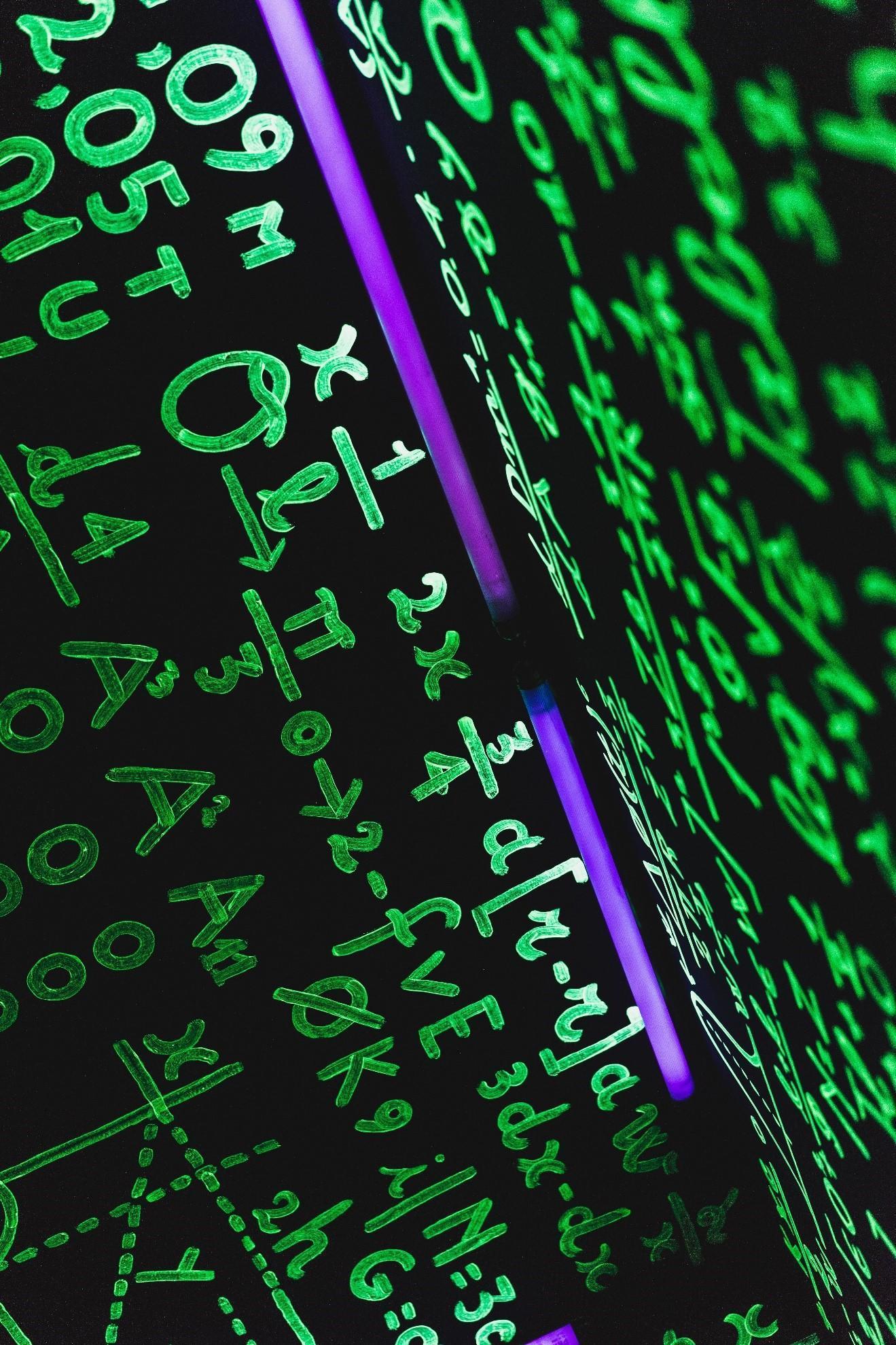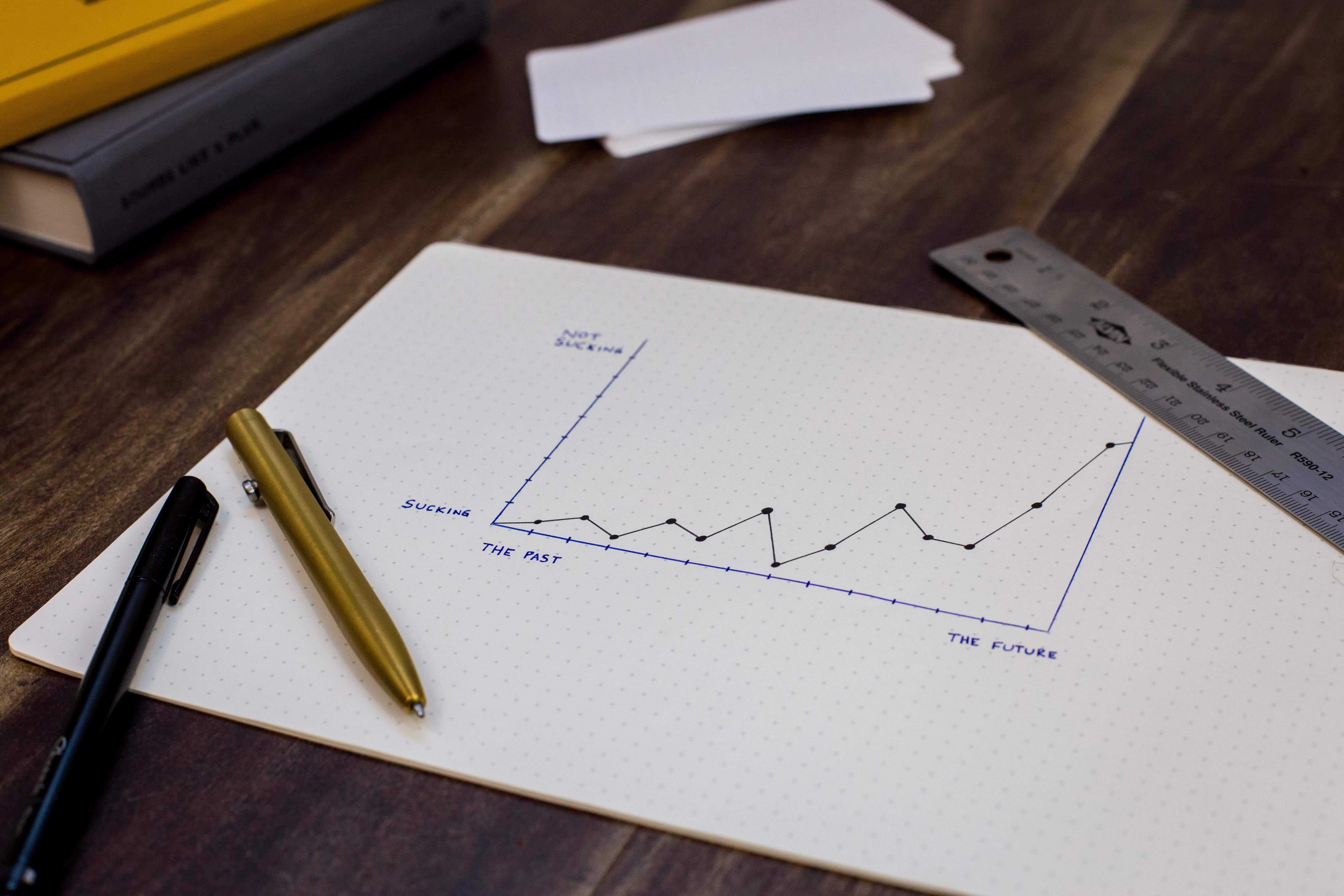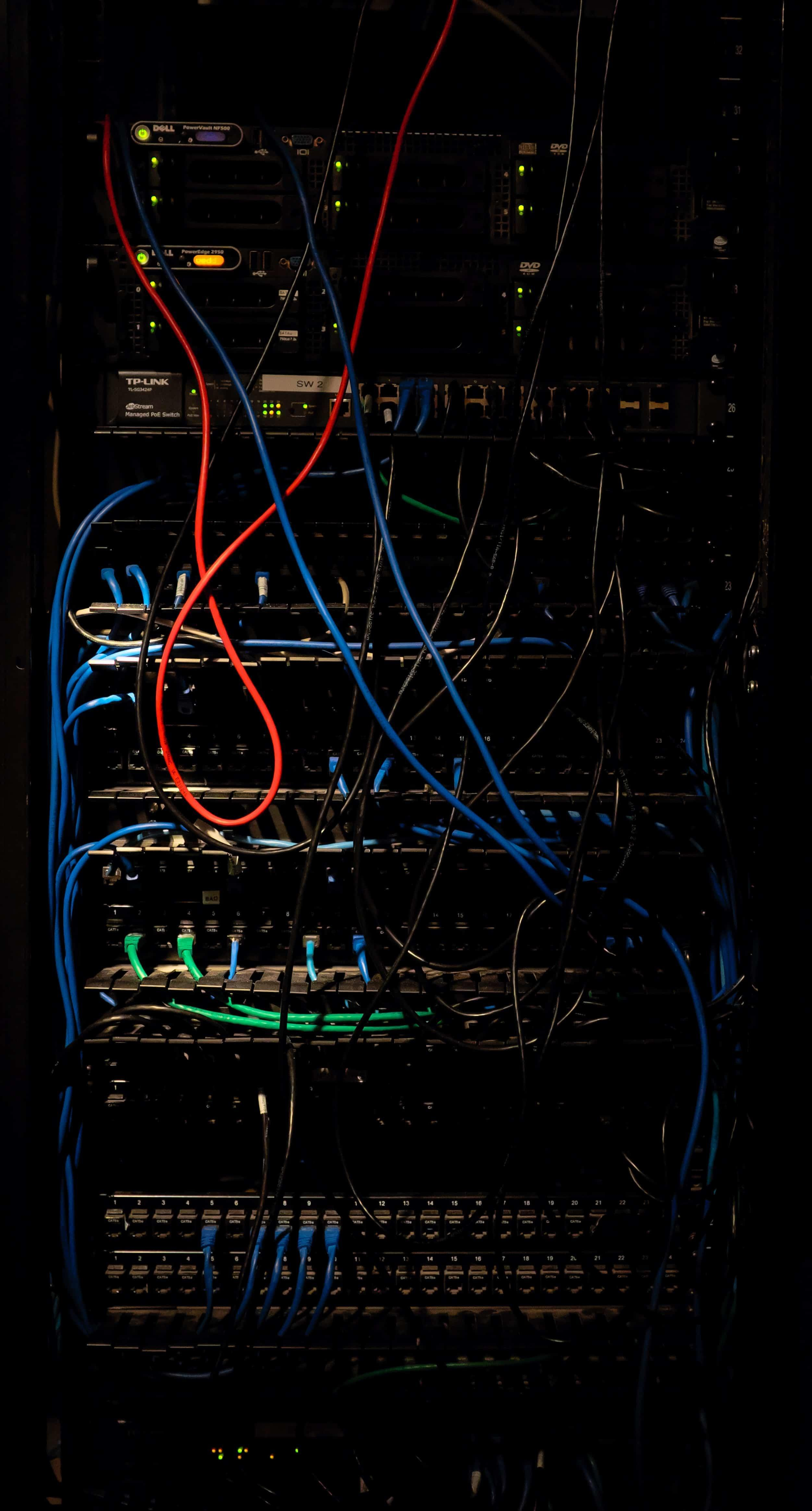Chapters

Statistics is one of those disciplines everyone knows at least a bit about. From interpreting a percentile to realizing a chi-square test, the broad discipline of statistics can be applied to every aspect of our lives: politics, romance, business and more. While the evolution of data analysis has a long history, the basics can be easy to grasp. Here’s everything you should know about statistical inference!Knowing key statistical functions can aid in learning new computer languages!The best Data Analysis tutors available
1st lesson is free!5 (3 reviews)
Merouane
\$20
/h
1st lesson is free!4.9 (7 reviews)
Utsav
\$30
/h
1st lesson is free!5 (7 reviews)
Neerja
\$25
/h
1st lesson is free!5 (3 reviews)
Yasmine
\$35
/h
1st lesson is free!5 (3 reviews)
Andrea
\$70
/h
1st lesson is free!Ashit
\$16
/h
1st lesson is free!5 (3 reviews)
Simran
\$18
/h
1st lesson is free!5 (1 reviews)
Ramandeep
\$15
/h
1st lesson is free!5 (3 reviews)
Merouane
\$20
/h
1st lesson is free!4.9 (7 reviews)
Utsav
\$30
/h
1st lesson is free!5 (7 reviews)
Neerja
\$25
/h
1st lesson is free!5 (3 reviews)
Yasmine
\$35
/h
1st lesson is free!5 (3 reviews)
Andrea
\$70
/h
1st lesson is free!Ashit
\$16
/h
1st lesson is free!5 (3 reviews)
Simran
\$18
/h
1st lesson is free!5 (1 reviews)
Ramandeep
\$15
/h

## Statistics Basics

Data analysis and statistical methods have been dominating headlines lately. The reason can be found in the ever-increasing use of data in all aspects of our lives - from the groceries people buy to the dating apps they use. Included in fields like biostatistics and business analytics, statistical data and statistical inference have actually been around long before the invention of computers. The statistician of the ancient world used both categorical and numerical data to record and analyse movements in agriculture, weather and commerce. While Bayesian statistics has revolutionized the work of statisticians to include more sophisticated methods of making predictions, statistics in the modern world has kept the three main essentials that started the discipline:

• Collecting data with a sample size
• Analysing the data
• Using creative ways of displaying or disseminating the conclusions from this data

## Statistical Computing for Beginners

While the intricacies of statistical analyses might seem too complicated for the layperson to grasp, even the most seasoned statistician or data scientist needs the occasional refresher on all things probability and statistics. Understanding the entirety of statistical techniques statistical theory into a couple of paragraphs can seem impossible, especially if you’re not too confident in your abilities in mathematical statistics. However, statistical data analysis is something you utilize on the daily: from crafting your monthly budget to creating insightful data visualizations at work. To start unpacking the world of statistical analysis we’ll have to start by making the important distinction between the two main branches of statistics: inferential and descriptive statistics. While descriptive statistics are used to describe and measure what is actually in your raw data, inferential statistics are used in order to make useful predictions about the general population using your sample data. Inferential statistics, on the other hand, tests a hypothesis and a null hypothesis on sample data in order to estimate metrics we can’t actually measure in real life, such as the true, population mean. Or, in other words, inferential statistics uses a set of data to make predictions about things outside that very data. Whether it be quantitative data or qualitative data, inferential statistics is one or the most important tools for data scientists the world over. It makes use of concepts such as probability theory and methods such as linear regression in order to make helpful predictions about the world. Go through these stand-out statistics courses here.The best Data Analysis tutors available
1st lesson is free!5 (3 reviews)
Merouane
\$20
/h
1st lesson is free!4.9 (7 reviews)
Utsav
\$30
/h
1st lesson is free!5 (7 reviews)
Neerja
\$25
/h
1st lesson is free!5 (3 reviews)
Yasmine
\$35
/h
1st lesson is free!5 (3 reviews)
Andrea
\$70
/h
1st lesson is free!Ashit
\$16
/h
1st lesson is free!5 (3 reviews)
Simran
\$18
/h
1st lesson is free!5 (1 reviews)
Ramandeep
\$15
/h
1st lesson is free!5 (3 reviews)
Merouane
\$20
/h
1st lesson is free!4.9 (7 reviews)
Utsav
\$30
/h
1st lesson is free!5 (7 reviews)
Neerja
\$25
/h
1st lesson is free!5 (3 reviews)
Yasmine
\$35
/h
1st lesson is free!5 (3 reviews)
Andrea
\$70
/h
1st lesson is free!Ashit
\$16
/h
1st lesson is free!5 (3 reviews)
Simran
\$18
/h
1st lesson is free!5 (1 reviews)
Ramandeep
\$15
/h

## Descriptive Statistics

Before getting into the exciting world of central tendency, ordinal data regression models, let’s start by looking at the most common tools used in exploratory analysis. In statistics, data can be analysed through univariate or multivariate methods, which roughly translates into analysing either one variable or multiple. Typically, univariate methods of analysis are more meaningful in initial exploratory analysis, where looking at and comparing the measures of specific variables can serve to highlight important features about your data set. While not at all an exhaustive explanation of descriptive statistics, here are some of the basics you can implement in your study design in order to understand your dependent or independent variable. Measures of central tendency, or what the average data looks like, are the sample mean, effect size, median and mode. Measures of variability, on the other hand, seek to measure how far data are spread from the average and include: variances, covariance and standard deviation. While this may sound very elementary, many industries that utilize statistics don’t need the more complex methods involved in inferential statistics. For example, using data visualizations of descriptive statistics, such as a histogram or pie chart, can help a company identify their biggest cost problems or the characteristics of an average client. In this way, exploratory analysis can turn into a powerful tool for both data visualization and analysis.Statistical analysis can be extremely helpful for all types of decision makers

## Inferential Statistics

Moving onto the concepts even the most seasoned mathematician can shudder at: inferential statistics. While more detailed explanations on some of the more complex statistical topics included under this branch of statistics, such as regression analysis with categorical data or binomial distributions, the basics are fairly easy to grasp. Underlying all inferential statistics is probability theory. From constructing a confidence interval for your estimators to attaining the statistical significance for a dependent variable – virtually all statistical methodology relies on probability theory. That being said, when it comes to inferential statistics, statisticians generally tend to be divided into two camps: frequentists and Bayesian(ists). While frequentists believe that probability is the measure of the frequency of an outcome from an actual trial, or experiment, the Bayesian statistician holds that probability is abstract and measures the belief in knowledge or proposition. If this sounds like someone just back-translated wingdings, it can be helpful to look at how a normal probability distribution is used in the most basic statistical model: linear regression analysis. In order to conduct linear regression analysis, as with many other types of analysis such as analysis of variance (ANOVA) or time series analysis, assumptions are made about the data in order to ensure the validity of the analysis. One of the most common assumptions is that the variables or the error term of the data follow a normal distribution. This ensure the validity of the model and is the basis of correct interpretation of everything from an alternative hypothesis and correlation coefficient to the estimator and confidence intervals. While most statistical software like R and SPSS will run statistical models automatically, it’s always important to check most assumptions of your data before running linear regression and other types of analysis. To give you an idea of what some other assumptions can look like, we can look at the Gauss-Markov theorem: If your linear regression model meets the first six classical assumptions of ordinary least squares method, then the regression is BLUE or the best linear unbiased estimator. Not only is the acronym easy to remember, but it also serves to underscore the important fact that any data meeting these assumptions can produce estimators with the least variance of all the possible estimators. The only downside, however, is that these assumptions can hardly ever be met in real life. Take a look and you’ll probably see why:

1. The model is linear in terms of both coefficients and the error term
2. The expected value, or mean, of the error term is zero
3. The independent variables are uncorrelated with the error term
4. There is no correlation between different observations of the error term
5. No heteroscedasticity in the error term, which can also be seen as constant variance
6. No perfect correlation between the independent variables

Another one of the more common statistical models you’re likely to find within any experimental design is the General Linear Model. This model is, at its most basic, a simple linear model and - among its most complex, can be used in multivariate analysis methods such as factor analysis, cluster analysis and more. Without getting into too much detail, using the GLM method for analysing both categorical and numerical data makes use of important concepts like the t-test to aid in determining the best model for the data. The t-test, at its base, assess whether the means of two groups are statistically different from one another and can be applied to make inferences on whether one linear model is better than another.From the hardware to the programs on a computer, statistics is involved!

## Resources for Statisticians

From the randomization of trials to the analysis of parametric models, creating the perfect methodology, analysis and interpretation in statistics can be tricky. Luckily, there are a number of ways you can get statistics help either online or with a professional. Whether you’re confused on the definition of a random variable, outliers, or observational data, the internet can be your best tool for finding statistics solutions. Check out Stack Exchange if you’re having trouble on a particular concept or problem.

### Where to Find Stats Tutors

If you’re looking for one-to-one statistics tutoring, make sure to browse through Superprof’s community of almost 150,000 maths tutors in the UK! Giving advice on everything related to mathematical statistics, you'll be able to receive advice and guidance on some of statistic's most troubling concepts and functions for the average price of 10 pounds an hour!

Need a Data Analysis teacher?

Enjoyed this article?5.00/5 - 1 review(s)Loading...Vanessa